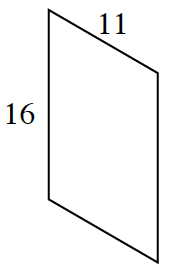### Home > INT2 > Chapter 3 > Lesson 3.2.4 > Problem3-110

3-110.Rakisha is puzzled. She is working with the parallelogram drawn at right and wants to make it smaller instead of bigger.

1. What should she do if she wants the sides of her new figure to be half as long as the original sides? What scale factor should she use? What are the dimensions of her new figure?

Rakisha should use a scale factor of $0.5$. The new side lengths will be $8$ and $5.5$.

2. While drawing some other shapes, Rakisha ended up with a shape congruent to the original parallelogram. What is the ratio between the pairs of corresponding sides?

What would Rakisha multiply her first shape's sides by to get the second shape?

The ratio is $1:1$ because the shapes are congruent.

3. Rakisha is convinced that every parallelogram with adjacent sides that measure $11$ and $16$ units will be congruent to the parallelogram above. Is she correct? Justify your answer using a proof or a counterexample.